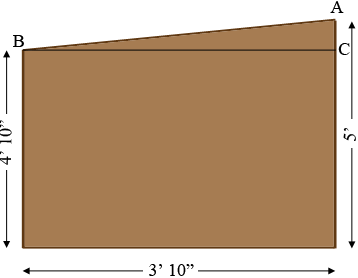SEARCH HOMEMath Central Quandaries & QueriesQuestion from david: Hi, I'm building some beagle kennels and I am in need of help with an angle problem. I need to place a roof on my kennel with a drop of 2inches across 3ft 10inches. the posts on the right side will be 5ft and the post on the left will be 4ft 10in. the posts are 4x4 and the space to be covered is 3ft 10in from the outside of the 4x4. Please help, thanks.Hi David,

In my diagram the triangle ABC has the side CA of length 2 inches and the side BC of length 3 feet 10 inches which is $3 \times 12 + 10 = 46$ inches.Thus

$\tan(CAB) = \frac{46}{2} = 23$

Thus the measure of the angle CAB is given by

$\tan^{-1}(23) = 87.5 \mbox{ degrees.}$

Hence you need to cut each of the posts with an angle measuring 87.5 degrees.

I hope this helps,
PennyMath Central is supported by the University of Regina and The Pacific Institute for the Mathematical Sciences.